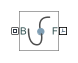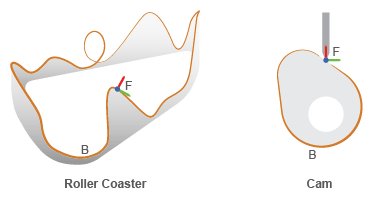# Point on Curve Constraint

Kinematic constraint between frame origin and curved path

•Libraries:
Simscape / Multibody / Constraints

## Description

The Point on Curve Constraint block confines a point to a curve. The frame attached to the point is free to rotate depending on other constraints in the model. Use the Point on Curve Constraint block to model point-on-curve constraints, such as that between a roller coaster and a track or a cam follower and a cam.The Point on Curve Constraint block has two ports: F and B. Port F is a frame port whose origin corresponds to the point. Port B is a geometry port that you connect to a curve. Avoid curves with sharp changes in slope, as these can cause simulation issues.

You can use this block to sense the constraint forces and torques between the point and curve. Based on the setting of the Resolution Frame parameter, the block resolves the forces and torques in either the base or follower frame.

## Ports

### Geometry

expand all

Geometry port associated with the curve defined by the Spline block.

### Frame

expand all

Follower frame whose origin is confined to the curve connected to port B.

### Output

expand all

Constraint force that acts between the point and curve, returned as a vector. See Measure Joint Constraint Forces for more information. If you set the Resolution Frame parameter to `Base`, the sensed force is with respect to the reference frame of the curve connected to port B.

#### Dependencies

To enable this port, under Constraint Force/Torque Sensing, select Force Vector.

Constraint torque that acts between the point and curve, returned as a vector. See Force and Torque Sensing for more information. If you set the Resolution Frame parameter to `Base`, the sensed torque is with respect to the reference frame of the curve connected to the port B.

#### Dependencies

To enable this port, under Constraint Force/Torque Sensing, select Torque Vector.

## Parameters

expand all

Direction in which to measure the constraint force and torque. Reversing this direction changes the sign of the measurements. See Force and Torque Measurement Direction for more information.

Frame used to resolve the measured vector quantities. See Selecting a Measurement Frame for more information.

Select this parameter to expose port f, which outputs the constraint force vector between the B and F frames.

Select this parameter to expose port t, which outputs the constraint torque vector between the B and F frames.

## Version History

Introduced in R2015b